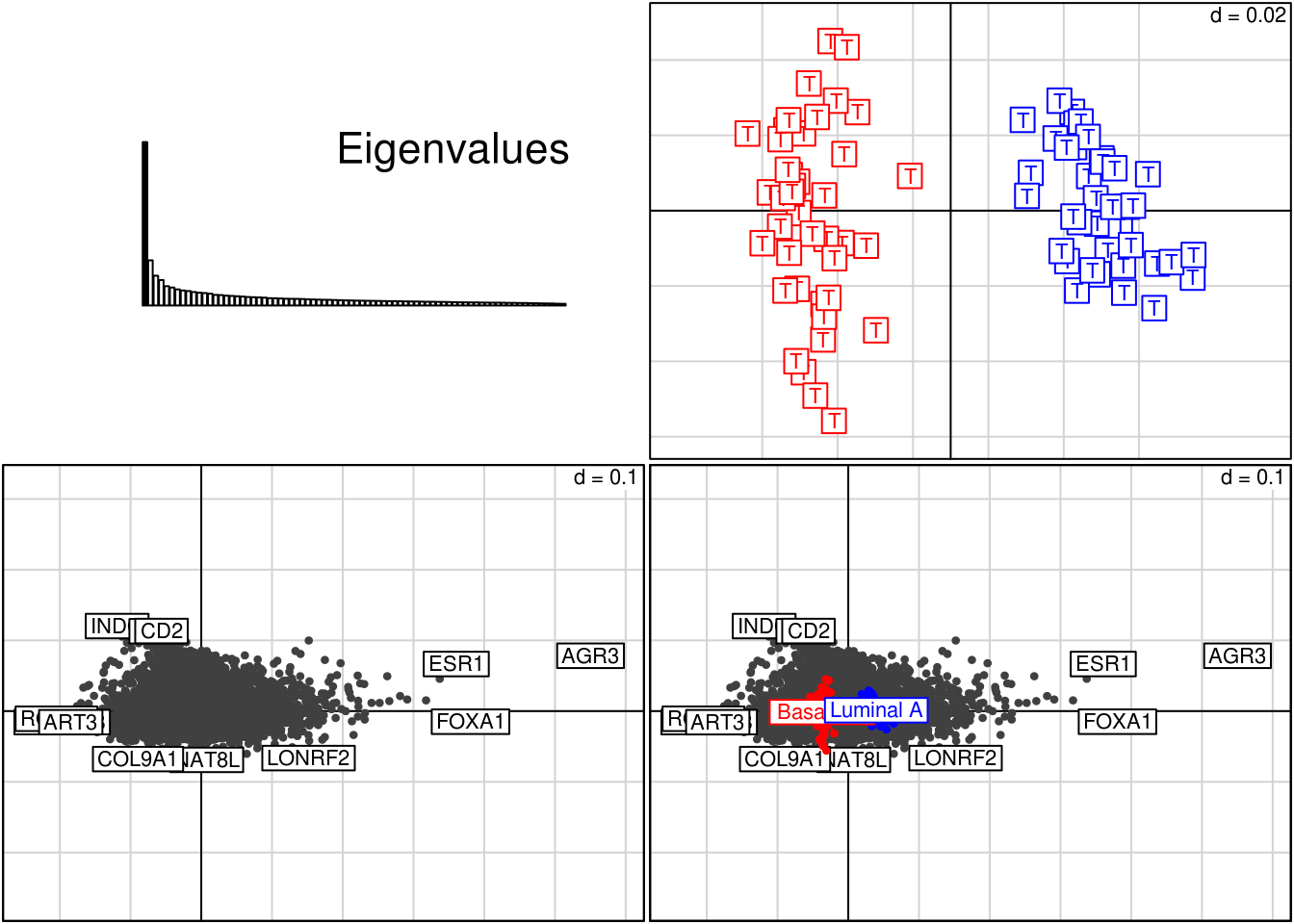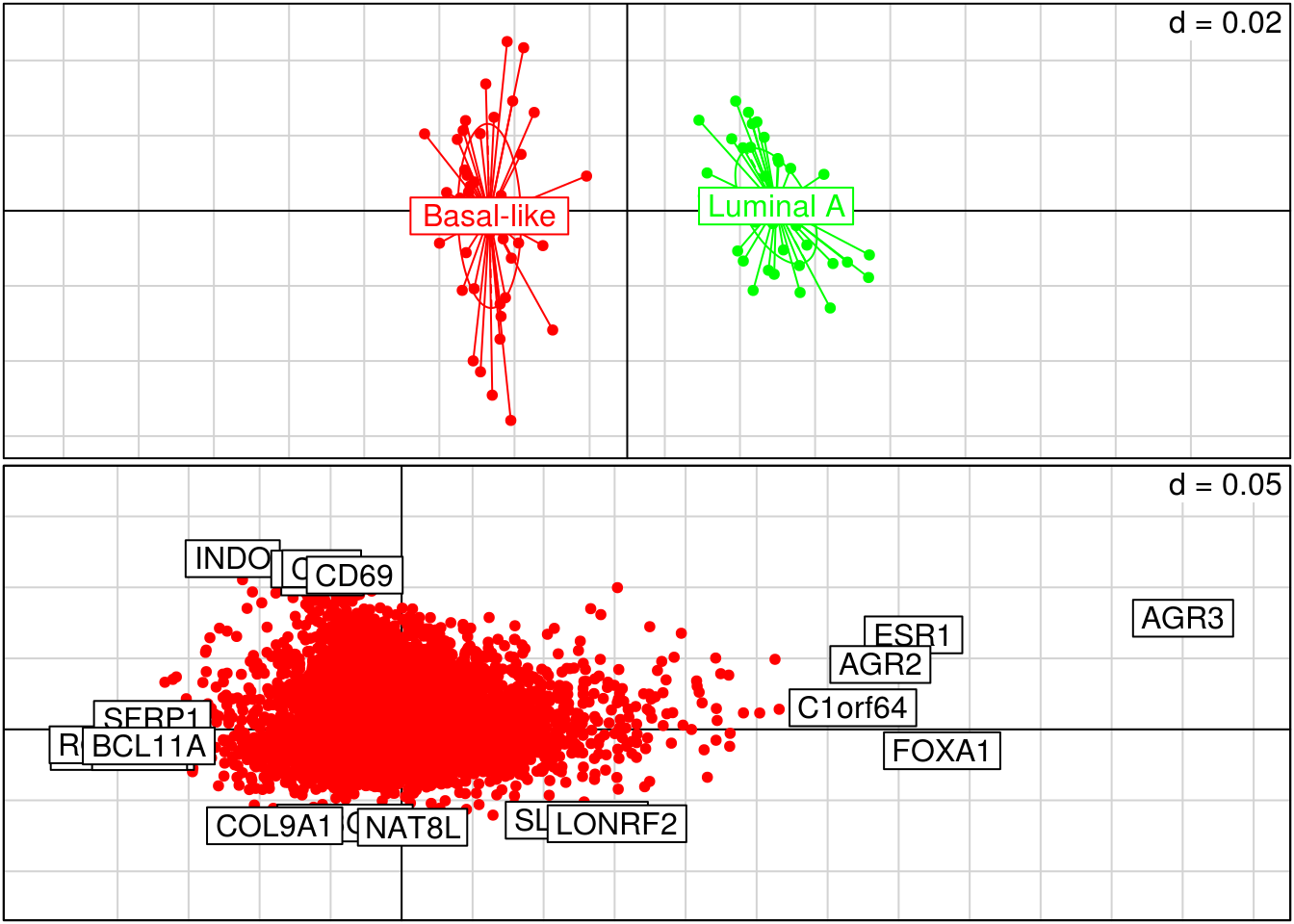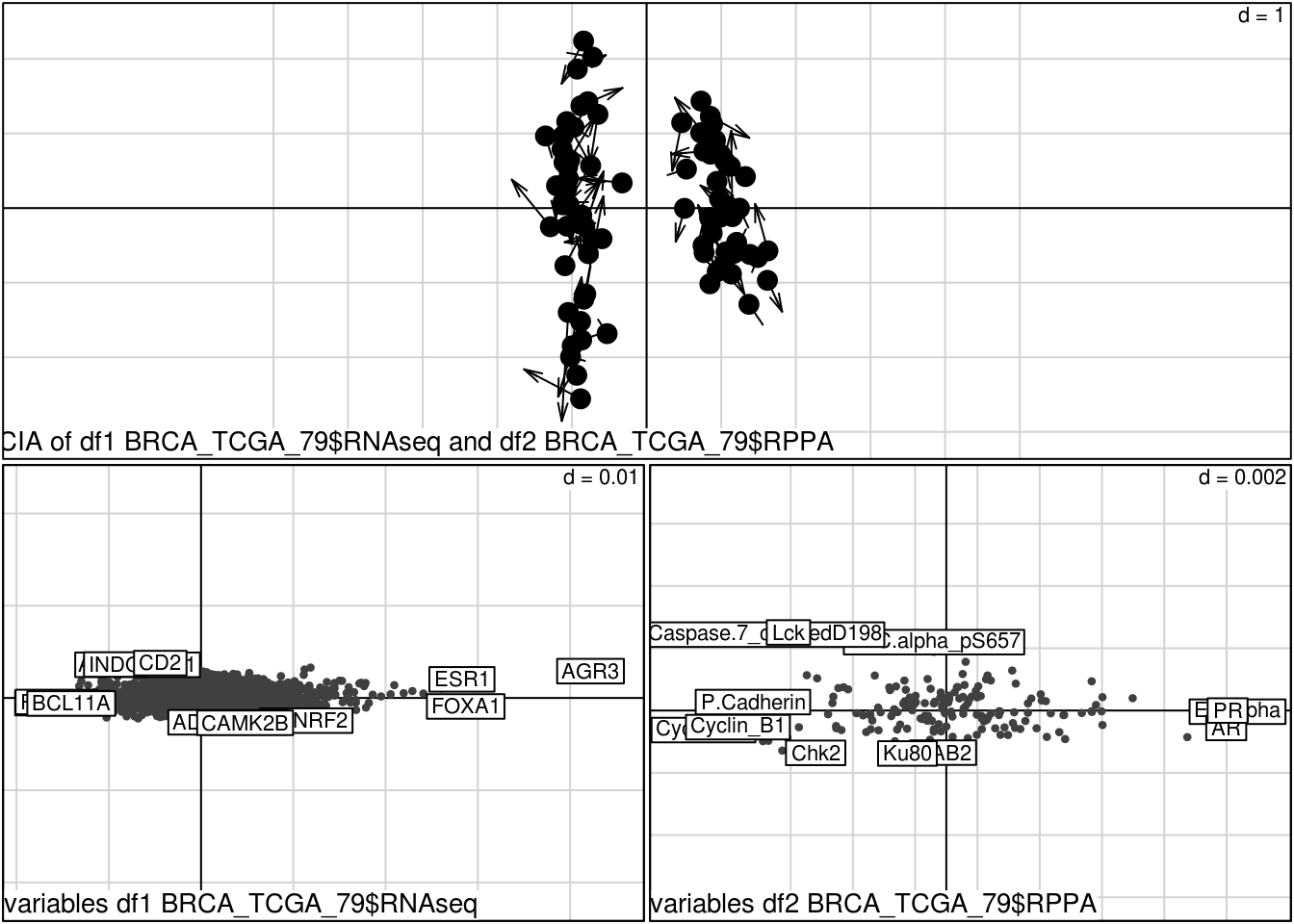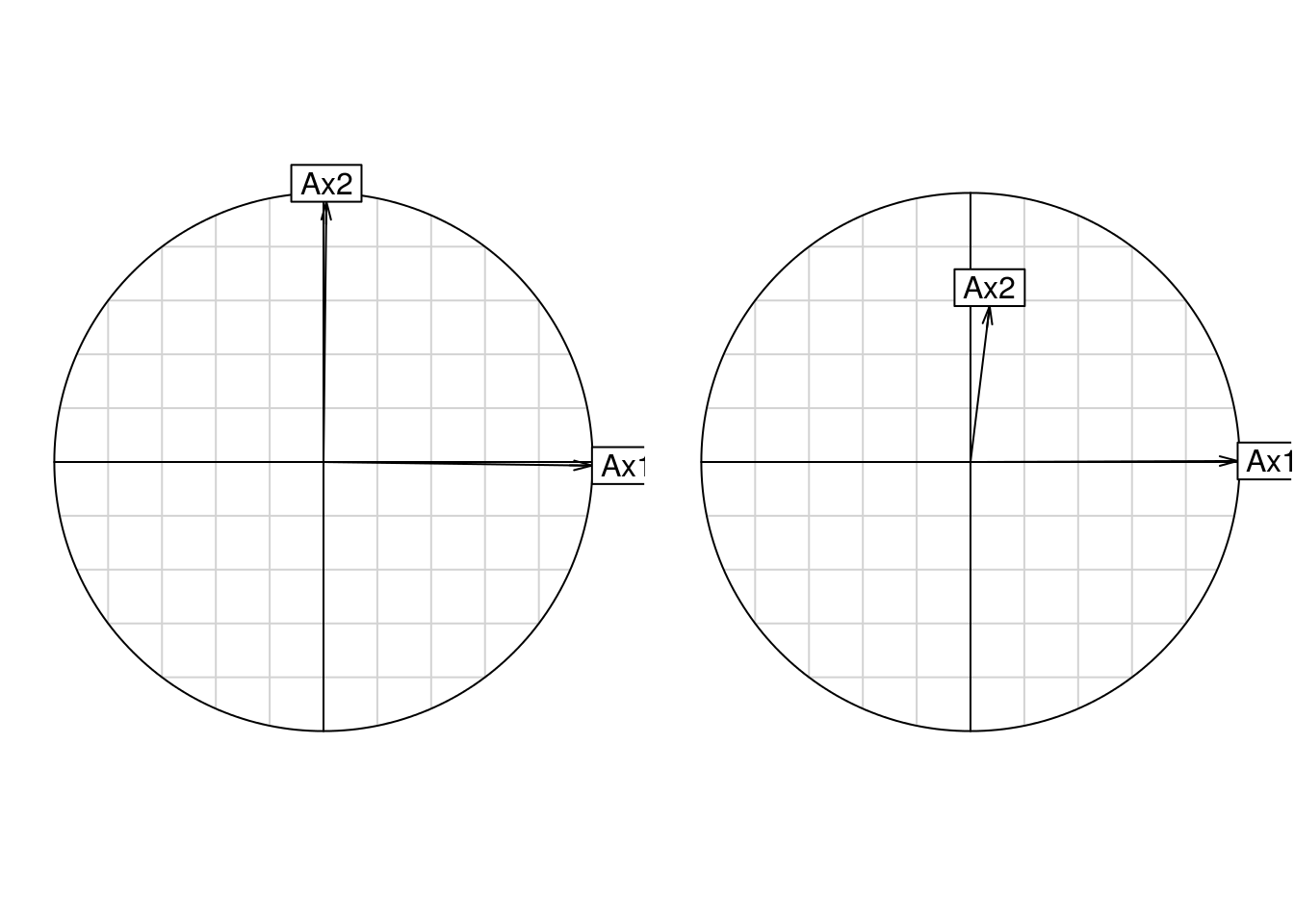## Introduction

Rapid advances and reducing costs have enabled laboratories to apply a multi-omic approach to biological systems. Studies frequently measure several biological molecules, including mRNA, proteins, metabolites, lipids and phosphoproteins using different technological platforms generating multiple datasets on the same set of biological samples. We have described the following different exploratory data analysis methods that enable one to identify co-relationships between high dimensional datasets.

• Multiple Co-Intertia Analysis (MCIA) simultaneously projects several datasets into the same dimensional space, transforming diverse sets of features onto the same scale and allows one identify the co-structure between datasets (Meng, Kuster, Culhane, and Moghaddas Gholami (2014)).

• iBBiG is a biclustering algorithm which we wrote for analysis of noisy binary data (Gusenleitner, Howe, Bentink, Quackenbush, and Culhane (2012)). We developed it to identify biclusters in results from GSEA/pathway analysis from >1,000 different studies. Taking several thousand vectors which each contain p-values enrichment scores for many thousands of pathways, we discretize these to create a binary matrix and apply iBBiG to find pathways associated with covariates across many studies.

Today, I will provide an overview of the first MCIA.

## TCGA Breast Cancer Dataset

In this workshop we will examine data from the the Cancer Genome Atlas (TCGA) Breast Cancer project (TCGA (2012)). We prepared a subset of the TCGA breast cancer study from the Nature 2012 publication. These are contained in a list and are multiple molecular data of 79 primary breast tumors. These data were downloaded from https://tcga-data.nci.nih.gov/docs/publications/brca_2012/. The data are miRNA, miRNAprecursor, RNAseq, Methylation, proteins measures on an RPPA array, and GISTIC SNP calls. I split the latter GISTIC data into 2 datasets; CNA and LOH (loss of heterzygousity), as these may be dervived by different biological mechanism and represent distinct molecular events in the cell (Wang, Birkbak, Culhane, Drapkin, Fatima, Tian, Schwede, Alsop, Daniels, Piao, and others (2012)). The eighth data.frame is the clinical data on these 79 cases. These data are in matrix or data.frame format.

(Note: more recently I have been using TCGA Assembler (Zhu, Qiu, and Ji (2014))) to download and process TCGA data. I am happy to describe this if there is interest.

I am also happy to review/demo how we reproduced the figures from our recent paper (Meng, Kuster, Culhane, et al. (2014)) and have uploaded code to regenerate the figures on the Bioconductor website.

``````load(file.path(datadir, "BRCA_TCGA_79_filtered_matchedNames_Basal+LuminalA.RData"))
summary(BRCA_TCGA_79)``````
``````##                Length  Class      Mode
## miRNA               79 data.frame list
## miRNAprecursor      79 data.frame list
## RNAseq         1055440 -none-     numeric
## Methyl              79 data.frame list
## RPPA                79 data.frame list
## LOH             996348 -none-     numeric
## CNA             934649 -none-     numeric
## clin                29 data.frame list``````

## Brief Background to ordination (PCA/COA)

MCIA can be computed using principcal component analysis (pca), correspondence analysis (coa) or one of several other ordination approaches.

There are many functions in Bioconductor and R for dimension reduction analysis. Within ade4 these are computed using the duality diagram (dudi) framework (Dray and Dufour (2007); De la Cruz and Holmes (2011)) and hence are called dudi.pca(), dudi.coa() etc.

``````library(ade4)
BRCApca<-dudi.pca(BRCA_TCGA_79\$RNAseq, scannf=FALSE, nf=5)
print(BRCApca)``````
``````## Duality diagramm
## class: pca dudi
## \$call: dudi.pca(df = BRCA_TCGA_79\$RNAseq, scannf = FALSE, nf = 5)
##
## \$nf: 5 axis-components saved
## \$rank: 79
## eigen values: 17.76 4.719 3.67 2.685 2.572 ...
##   vector length mode    content
## 1 \$cw    79     numeric column weights
## 2 \$lw    13360  numeric row weights
## 3 \$eig   79     numeric eigen values
##
##   data.frame nrow  ncol content
## 1 \$tab       13360 79   modified array
## 2 \$li        13360 5    row coordinates
## 3 \$l1        13360 5    row normed scores
## 4 \$co        79    5    column coordinates
## 5 \$c1        79    5    column normed scores
## other elements: cent norm``````

(In ade4, \$co are columns and \$li are lines or rows)

``summary(BRCApca)``
``````## Class: pca dudi
## Call: dudi.pca(df = BRCA_TCGA_79\$RNAseq, scannf = FALSE, nf = 5)
##
## Total inertia: 79
##
## Eigenvalues:
##     Ax1     Ax2     Ax3     Ax4     Ax5
##  17.763   4.719   3.670   2.685   2.572
##
## Projected inertia (%):
##     Ax1     Ax2     Ax3     Ax4     Ax5
##  22.485   5.973   4.646   3.398   3.256
##
## Cumulative projected inertia (%):
##     Ax1   Ax1:2   Ax1:3   Ax1:4   Ax1:5
##   22.49   28.46   33.10   36.50   39.76
##
## (Only 5 dimensions (out of 79) are shown)``````

We have a BioC packages called made4 which is a wrapper around the ade4 that utilizes Bioconductor data classes such as Expression Set. In made4 the simplest way to view results from the function ord is to use the function plot. This will draw a plot of the eigenvalues, along with plots of the variables (genes) and a plot of the cases (microarray samples).

``````library(made4)
cl<-as.character(BRCA_TCGA_79\$clin\$PAM50.mRNA)
BRCAord= ord(BRCA_TCGA_79\$RNAseq, classvec=factor(cl))
summary(BRCAord\$ord)``````
``````## Class: coa dudi
## Call: dudi.coa(df = data.tr, scannf = FALSE, nf = ord.nf)
##
## Total inertia: 0.006782
##
## Eigenvalues:
##       Ax1       Ax2       Ax3       Ax4       Ax5
## 0.0015441 0.0004271 0.0002812 0.0002410 0.0001826
##
## Projected inertia (%):
##     Ax1     Ax2     Ax3     Ax4     Ax5
##  22.767   6.297   4.146   3.553   2.692
##
## Cumulative projected inertia (%):
##     Ax1   Ax1:2   Ax1:3   Ax1:4   Ax1:5
##   22.77   29.06   33.21   36.76   39.45
##
## (Only 5 dimensions (out of 78) are shown)``````
``plot(BRCAord, nlab=3,   arraylabels=rep("T", 79))``figure 1: Correspondence analysis plots. A. plot of the eigenvalues, B. projection of microarray samples C. projection of genes (gray filled circles) and D. biplot showing both genes and samples. Samples and genes with a strong association are projected in the same direction from the origin. The greater the distance from the origin the stronger the association . The distinction between molecular subtypes is captured on the first eigenvector.

The case and gene projections can be visualised with functions plotarrays and plotgenes (sorry the names of these functions steam from the olden days of microarrays). These are wrappers around ade4 plotting functions. The number of genes that are labelled at the end of the axis can be defined. The default is 10. These plots are simplistic and could be rendered more useful with shiny/ggplots/etc

``````par(mfrow=c(2,1))
plotarrays(BRCAord\$ord\$co, classvec=BRCA_TCGA_79\$clin\$PAM50.mRNA)
plotgenes(BRCAord, n=5, col="red")``````Figure 2. Plot of A) sample coordinates b) feature coordinates

To extract a list of variables with greatest loadings or weights on an axes, (ie those at the end of an axes), use function topgenes. For example, to get a list of the 5 genes at the negative and postive ends of axes 1.

``ax1<- topgenes(BRCAord, axis=1, n=5)``

## CIA: Two datasets

Coinertia analysis (CIA) identifies trends or co-relationships in two datasets which contain the same samples. Either the rows or the columns of a matrix must be “matchable”. CIA can be applied to datasets where the number of variables exceed the number of samples.

CIA is related to canonical correlation analysis, but CIA optimizes the squared covariance between the eigenvectors whereas CCA optimizes the correlation. There was a nice comparison of sparse CCA and CIA by LeCao et al., LeCao2009 (Le Cao, Martin, Robert-Granie, and Besse (2009))

The function cia calls the function coinertia in the R package ade4 .

``````## Coinertia analysis
## call: coinertia(dudiX = coa1, dudiY = coa2, scannf = cia.scan, nf = cia.nf)
## class: coinertia dudi
##
## \$rank (rank)     : 78
## \$nf (axis saved) : 2
## \$RV (RV coeff)   : 0.8315
##
## eigenvalues: 9.302e-06 4.838e-07 2.381e-07 2.02e-07 1.475e-07 ...
##
##   vector length mode    content
## 1 \$eig   78     numeric Eigenvalues
## 2 \$lw    171    numeric Row weigths (for coa2 cols)
## 3 \$cw    13360  numeric Col weigths (for coa1 cols)
##
##    data.frame nrow  ncol  content
## 1  \$tab       171   13360 Crossed Table (CT): cols(coa2) x cols(coa1)
## 2  \$li        171   2     CT row scores (cols of coa2)
## 4  \$co        13360 2     CT col scores (cols of coa1)
## 5  \$c1        13360 2     Principal axes (cols of coa1)
## 6  \$lX        79    2     Row scores (rows of coa1)
## 7  \$mX        79    2     Normed row scores (rows of coa1)
## 8  \$lY        79    2     Row scores (rows of coa2)
## 9  \$mY        79    2     Normed row scores (rows of coa2)
## 10 \$aX        2     2     Corr coa1 axes / coinertia axes
## 11 \$aY        2     2     Corr coa2 axes / coinertia axes
##
## CT rows = cols of coa2 (171) / CT cols = cols of coa1 (13360)``````figure 4. Plot of coinertia analysis of gene and protein expression datasets.

The correlation between the coa axes and the coinertia axes are in \(aX and \)aY:

``````par(mfrow=c(1,2))
s.corcircle(BRCAcia\$coinertia\$aX)
s.corcircle(BRCAcia\$coinertia\$aY)``````fig 5 Scatter diagram of a correlation circle of the coa and coinertia axes. The angle between two vectors gives the degree of correlation (adjacent = highly correlated, orthogonal (90°) = uncorrelated, and opposite (180°) = negatively correlated).

The RV coefficient is a measure of global similarity between the datasets. The RV coefficient of this coinertia analysis is: 0.8315.

## MCIA

Multiple Co-Interia Analysis (MCIA) simultaneously projects several datasets into the same dimensional space, transforming diverse sets of features onto the same scale, enabling one to extract the most variant from each dataset (Meng, Kuster, Culhane, et al. (2014)).

``````# Check that there are no zero or low variant low count rows.
for (i in 1:7) BRCA_TCGA_79[[i]]<-BRCA_TCGA_79[[i]][!apply(BRCA_TCGA_79[[i]],1, sum)==min(BRCA_TCGA_79[[i]]),]

``plot(mcia79, axes=1:2, sample.lab=FALSE, sample.legend=FALSE, phenovec=as.numeric(BRCA_TCGA_79\$clin\$PAM50.mRNA), gene.nlab=2, df.color=c("navy", "cyan", "magenta", "red4", "brown","yellow", "orange"),df.pch=2:8)``